• 本文通过python实现影响力最大化模型，所用例子为空手道俱乐部。
本文通过python实现影响力最大化模型，所用例子为空手道俱乐部。
文章目录： 社交网络分析——信息传播模型（附带三个模型的python实现） 信息传播模型——SIR的Python实现
大纲：
什么是影响力衡量影响力影响力最大化问题
影响力定义： 影响力，一般认为指的是用一种为别人所乐于接受的方式，改变他人的思想和行动的能力。(搜狗百科)
影响，控制或操纵某物或某人的权力； 改变诸如行为，思想或决定之类的波动事物发展的能力。
影响扩散的因素
网络结构（无权） 密度 度分布 连通区域 社区结构链接强度（有权） 交流频率 影响力信息质量 信息的吸引力和特殊性
影响力最大化 问题：选择k个种子用户去最大化影响用户数量 输入：具有边影响概率的社交网络图 输出：大小为k的种子集合
问题陈诉：
集合S的扩散：F(S) 如果集合S为初始集合，则结果为期望的激活节点的数量；问题： 给定一个参数k，找到一个节点数为k的集合S来最大化F(S) 以F(S)为目标函数的约束优化问题
F(S)特性： 非负的 单调的：F(S+v)≥F(S)
代码：
import networkx as nx
import numpy as np
import random

'''
author：xiao黄
time：2020.10.23
blog：https://blog.csdn.net/Python_Matlab
'''

# 计算节点集的影响力
def influence_computation_IC(seed):
influence = 0
if len(seed) == 0: # 种子为0 直接返回0 -> 无影响力
return influence

for i in range(R):
result_list = []
result_list.extend(seed)

# 保存激活的状态
checked = np.zeros(g.number_of_nodes())
for node in result_list:
checked[node] = 1

# 当前节点不为空，进行影响
while len(result_list) != 0:
influence += 1
current_node = result_list.pop(0)
for nbr in g.neighbors(current_node): # 得到当前节点的邻居节点
if checked[nbr] == 0:
wt = g.get_edge_data(current_node, nbr)
if random.uniform(0,1) < wt['weight']:
checked[nbr] = 1
result_list.append(nbr)

return influence/R

if __name__ == "__main__":
k = 3 # 影响力最大的几个点，这里设置为三个点
R = 1000 # 传播过程 一般 10000次
seed = []
node_list = []

g = nx.karate_club_graph() # 空手道俱乐部

for edge in g.edges:

for i in range(k):
f = np.zeros(g.number_of_nodes())
state = np.zeros(g.number_of_nodes())
for v in seed:
state[v] = 1
for v in g.nodes:
node_list.extend(seed)
if state[v] == 0:
node_list.append(v)
f[v] = influence_computation_IC(node_list) - influence_computation_IC(seed)
node_list.clear()
print(f)
seed.append(f.argmax())
print(seed)

运行结果：
[25.077 24.788 25.167 25.008  9.963 12.557 13.154 21.757 24.127 23.861
10.47  13.772 21.72  25.032 16.781 22.805 12.536 17.224  3.04  23.631
5.204 15.163 24.503 25.039 15.542 18.434 23.283 13.992 18.303 24.907
23.444 24.781 25.    25.121]
[ 0.184 -0.157  0.     0.348  1.339  1.693  1.554  0.178  0.17   0.032
1.177  0.424  0.308  0.216  0.725  0.135  1.614  0.228  0.97   0.375
0.7    0.275  0.284  0.385  0.814  0.704  0.391  1.007  0.45   0.384
-0.121  0.389  0.17   0.387]
[-0.086  0.142  0.     0.102  0.759  0.     0.267  0.18   0.099 -0.091
0.734  0.282  0.199  0.203  0.57   0.277 -0.017  0.374  0.906  0.45
0.762  0.555  0.232  0.237  0.95   0.337  0.326  0.863  0.487  0.284
0.141  0.318  0.234  0.24 ]
[2, 5, 24]

其中[2,5,24]为某次运行得到的影响力最大化的三个节点。
注意：因为本文中边的权值是随机的，所以每次运行结果可能不一样。
展开全文python 社交网络 复杂网络
• 文章目录影响力最大化RIS算法简介随机反向可达集最大贪婪覆盖算法算法近似比时间复杂度源代码 影响力最大化RIS算法 简介 对于影响力最大化问题，我以前写过几个blog 影响力最大化 IC模型+贪心算法 影响力最大化 模拟...


文章目录
影响力最大化RIS算法简介随机反向可达集最大贪婪覆盖算法算法近似比时间复杂度源代码

影响力最大化RIS算法
简介
对于影响力最大化问题，我以前写过几个blog 影响力最大化 IC模型+贪心算法 影响力最大化 模拟爆发(粗糙笔记) 影响力最大化 IC 蒙特卡洛模拟 贪心算法 影响力最大化 IMRank 我心中的最优算法 影响力最大化 CELF 成本效益延迟转发算法
这篇文章主要是介绍一种解决静态网络的影响力最大化问题，RIS方法是Reverse Influence Sampling，反向影响力采样，这是我直译的，但是这个中文名字其实很真实的反映了这个算法的几个重点思想，在后面我们一一介绍。
RIS算法是2014年提出的，感兴趣的话大家可以去scholar搜一下。算是比较新的IM算法了。
随机反向可达集
我们用一个示例来进行展示，首先创建这样的一个图：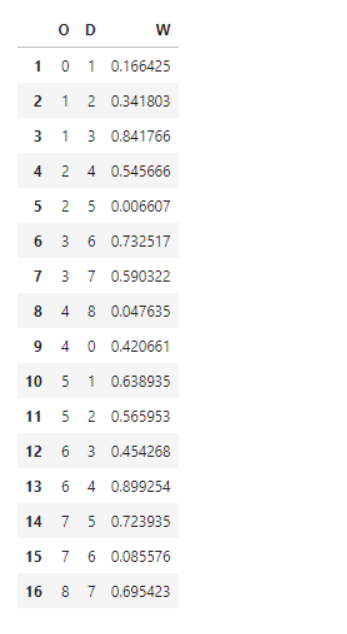打印出来是这样的：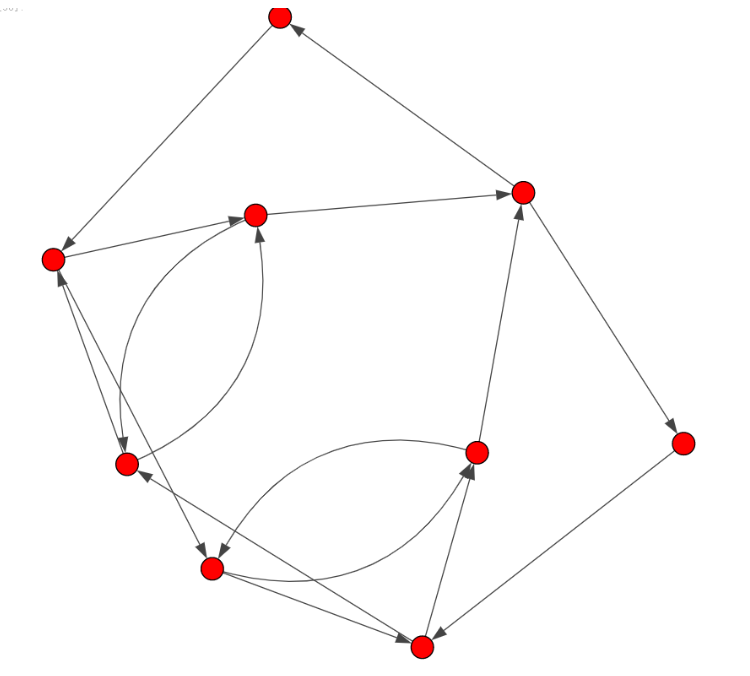然后对上图进行采样，也就是生成一个01之间的随机数，如果某一条边比这个随机数高的话那么这条边就保留，否则就去掉。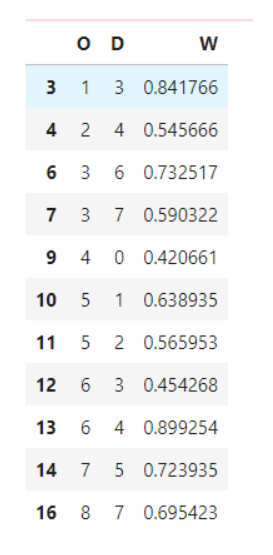于是我们得到这样的图：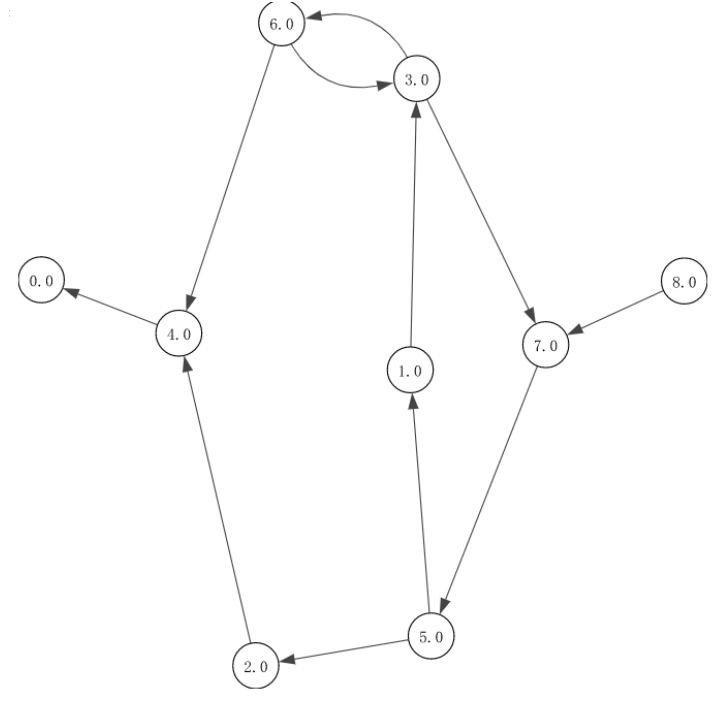这就完成了一次RIS采样。然后我们这样定义随机反向可达集（Random Reverse Reachable Set，以下称为RRS）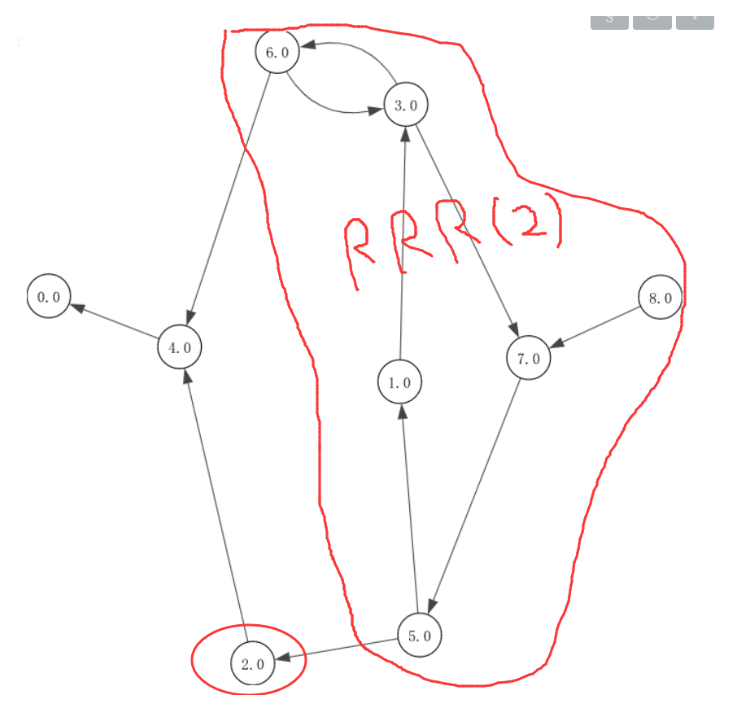如上图所示，我们求的是v2的RRS，可见我们可以理解为，节点v的RRS中的任意节点u被激活都有可能激活节点v。RRS很好理解的。
关键就是如何求解RRS，在真正的应用中一般不会只求一个点的RRS，而是一个source点集的RRS。

求解RRS大致思路：
new_set = [source], RRR_tmp = [source]while(new_set 不为空) tmp = g[那些边的D是在source里面][‘O’] RRR = list(set(RRR_tmp + tmp)) new_set = list(set(RRS) - set(RRR_tmp)) RRR_tmp = RRR[:] return(RRR)

最大贪婪覆盖算法
其实这里不是要介绍这个算法，现在我们已经知道如何求解RRS了，接下来就需要用到最大贪婪覆盖算法来解决影响力最大化问题了。
首先我们不是要得到一个RRS，而是随机选择N个（一般N比较大，超过10000，越多正确率越高）source集合，然后得到N个RRS集合。在这N个RRS集合中我们使用最大贪婪算法：

RRS_list中有N个RRS
for i in range(k): #k表示要求解的seed集合中有几个元素。 寻找RRS_list中出现次数最多的v，添加到seed集合 将拥有v节点的RRS集合在RRS_list中删掉 return（seed）

在实现的代码中我使用的是词袋模型来找到出现次数最多的v。
算法近似比
在原来的博客中有朋友问到了近似比，原来一直没注意，我会在以后的图算法中增加这一个度量的分享。
很明显RIS算法得到的不是最优解，而是近似最优解，这时候就有一个重要的衡量：近似比。
假设一个最优化问题的最优解是c*，近似最优解是c，那么算法的近似比是：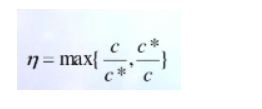通常情况下，这个性能近似比是输入规模n的函数：RIS的近似比： (1−1/e−ϵ)
时间复杂度
RIS的事件复杂度大大低于IC和CELF，这是有道理的。
我们可以这么想，CELF和IC都是通过贪心遍历然后得到局部最优解。随着n的增大事件复杂度暴增，这就是我觉得CELF和IC贪心是不太实用的传播模型。
但是RIS只在一开始进行一次生成RRS的遍历，然后在求解过程中并不存在后继的遍历计算。用论文中的一句话一开始生成的RRS用于通知网络内的节点扩展。
但是和IMRank算法的时间效率我不太确定，后面我会下一篇关于这两个的事件效率的比较。我先预测一下，我觉得IMRank会更快一些，但是随着n的增加不知道会呈现什么趋势。
源代码
传送门

大家共勉~

展开全文算法 python 图计算
• 社交网络影响力最大化——贪心算法实现（Python实现） １、环境配置 环境： Win7 Pycharm Anaconda2 该算法每个节点的阈值设为 0.5 ２、LT传播模型算法实现 linear_threshold_clime.py（LT传播模型算法） #...
目录
１、环境配置
２、LT传播模型算法实现
３、贪心算法实现
４、LT模型改进算法实现

社交网络影响力最大化——贪心算法实现（Python实现）
１、环境配置
环境： Win7 Pycharm Anaconda2
该算法每个节点的阈值设为 0.5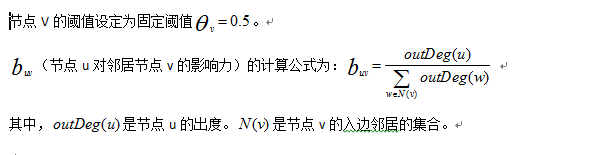２、LT传播模型算法实现
linear_threshold_clime.py（LT传播模型算法）
# -*- coding: utf-8 -*-
"""
Implement linear threshold models

"""
import copy
import itertools
import random
import math
import networkx as nx

__all__ = ['linear_threshold']

#-------------------------------------------------------------------------
#  Some Famous Diffusion Models
#-------------------------------------------------------------------------

def linear_threshold(G, seeds, steps=0):
"""

Parameters
----------
G : networkx graph
The number of nodes.

seeds: list of nodes
The seed nodes of the graph

steps: int
The number of steps to diffuse
When steps <= 0, the model diffuses until no more nodes
can be activated

Return
------
layer_i_nodes : list of list of activated nodes
layer_i_nodes: the seeds
layer_i_nodes[k]: the nodes activated at the kth diffusion step

Notes
-----
1. Each node is supposed to have an attribute "threshold".  If not, the
default value is given (0.5).
2. Each edge is supposed to have an attribute "influence".  If not, the
default value is given (out_deg / out_deg_sum)

"""
if type(G) == nx.MultiGraph or type(G) == nx.MultiDiGraph:
raise Exception( \
"linear_threshold() is not defined for graphs with multiedges.")

# make sure the seeds are in the graph
for s in seeds:
if s not in G.nodes():
raise Exception("seed", s, "is not in graph")

# change to directed graph
if not G.is_directed():
#DG = G.to_directed()
DG=nx.DiGraph(G)
else:
DG = copy.deepcopy(G)

# init thresholds
for n in DG.nodes():
if 'threshold' not in DG.node[n]:
DG.node[n]['threshold'] = 0.5
elif DG.node[n]['threshold'] > 1:
raise Exception("node threshold:", DG.node[n]['threshold'], \
"cannot be larger than 1")

# init influences

# in_deg_all = DG.in_degree()        #获取所有节点的入度
out_deg_all=DG.out_degree()        #获取所有节点的出度
in_edges_all=DG.in_edges()         #获取所有的入边
for e in DG.edges():               #对所有的边进行循环
if 'influence' not in DG[e][e]:
out_deg=out_deg_all[e]      #获取节点e的出度
edges_dict=dict(in_edges)
in_all_edges=list(edges_dict.keys())      #获取节点e的所有入边节点并存入列表
out_deg_sum=0
for i in in_all_edges:                #求节点e所有入边节点的出度和
out_deg_sum=out_deg_sum+out_deg_all[i]
DG[e][e]['influence'] = out_deg / out_deg_sum
elif DG[e][e]['influence'] > 1:
raise Exception("edge influence:", DG[e][e]['influence'], \
"cannot be larger than 1")

# perform diffusion
A = copy.deepcopy(seeds)
if steps <= 0:
# perform diffusion until no more nodes can be activated
return _diffuse_all(DG, A)
# perform diffusion for at most "steps" rounds only
return _diffuse_k_rounds(DG, A, steps)

def _diffuse_all(G, A):
layer_i_nodes = [ ]
layer_i_nodes.append([i for i in A])
while True:
len_old = len(A)
A, activated_nodes_of_this_round = _diffuse_one_round(G, A)
layer_i_nodes.append(activated_nodes_of_this_round)
if len(A) == len_old:
break
return layer_i_nodes

def _diffuse_k_rounds(G, A, steps):
layer_i_nodes = [ ]
layer_i_nodes.append([i for i in A])
while steps > 0 and len(A) < len(G):
len_old = len(A)
A, activated_nodes_of_this_round = _diffuse_one_round(G, A)
layer_i_nodes.append(activated_nodes_of_this_round)
if len(A) == len_old:
break
steps -= 1
return layer_i_nodes

def _diffuse_one_round(G, A):
activated_nodes_of_this_round = set()
for s in A:
nbs = G.successors(s)
for nb in nbs:
if nb in A:
continue
active_nb = list(set(G.predecessors(nb)).intersection(set(A)))
if _influence_sum(G, active_nb, nb) >= G.node[nb]['threshold']:
A.extend(list(activated_nodes_of_this_round))
return A, list(activated_nodes_of_this_round)

def _influence_sum(G, froms, to):
influence_sum = 0.0
for f in froms:
influence_sum += G[f][to]['influence']
return influence_sum


３、贪心算法实现
test_linear_threshold_clime.py
#!/usr/bin/env python
# coding=UTF-8
from nose.tools import *
from networkx import *
from linear_threshold_clime import *
"""Test Diffusion Models
----------------------------
"""
#贪心算法
def _linear_clime(G,k):      #参数k-表示要获取的子节点的个数
allnodes = G.nodes()     #获取图中所有节点数
seed_nodes = []          #该列表存储选取的子节点集
for m in range(k):
all_nodes = list(allnodes)   #将所有的节点存储在all_nodes列表里
layers_activite = []    # 存储每个节点的激活节点列表
lengths = []         # 存储每个节点的激活列表长度
datas = []
for i in all_nodes:   #遍历所有的节点，分别求出每个节点对应的激活节点集以及激活节点集的长度
data = []
data.append(i)
datas.append(i)
data_test=seed_nodes+data
layers = linear_threshold(G,data_test)
data.pop()
del layers[-1]
length = 0
layer_data = []
for j in range(len(layers)):
length = length + len(layers[j])
layer_data = layer_data + layers[j]
length_s = length - len(layers)
for s in range(len(layers)):
del layer_data
layers_activite.append(layer_data)
lengths.append(length_s)
# length_max = max(lengths)  # 获取激活节点最多的节点数
layers_max = layers_activite[lengths.index(max(lengths))]  # 获取被激活节点数最多的列表
seed_nodes.append(datas[lengths.index(max(lengths))])      # 获取被激活节点最多的子节点
for i in all_nodes:       #该循环是删除所有节点中seed_nodes节点集
if i in seed_nodes:
del all_nodes[all_nodes.index(i)]
allnodes=all_nodes
return seed_nodes,layers_max     #返回值是贪心算法求得的子节点集和该子节点集激活的最大节点集

#测试算法
if __name__=='__main__':
datasets=[]
f=open("Wiki-Vote.txt")
rows=data.split('\n')
for row in rows:
split_row=row.split('\t')
name=(int(split_row),int(split_row))
datasets.append(name)
G=networkx.DiGraph()

n=input('Please input the number of seeds: k=')
k=int(n)
seed_nodes, layers_max=_linear_clime(G,k)   #调用贪心算法获取节点子集和节点子集的最大激活节点集

print(seed_nodes)
print(layers_max)


运行结果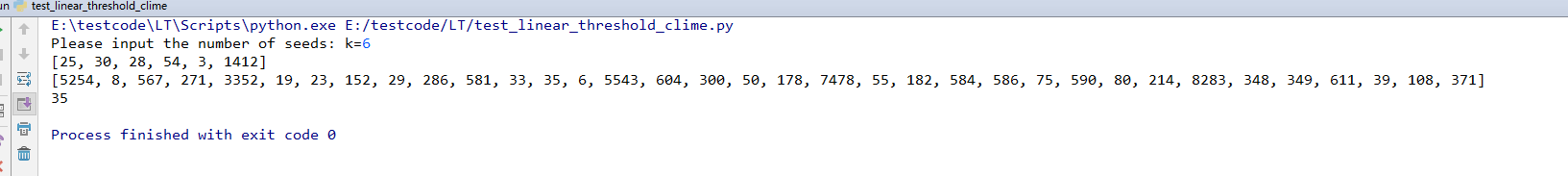４、LT模型改进算法实现
LT_improve.py（LT模型改进算法）
#!/usr/bin/env python
# coding=UTF-8
from nose.tools import *
from networkx import *
from linear_threshold_clime import *
from linear_threshold  import *
import math

#计算图中边的权重
def Buv_calculate(G,u,v):
out_deg_all = G.out_degree()  # 获取所有节点的出度
in_edges_all = G.in_edges()  # 获取所有的入边
out_deg = out_deg_all[u]  # 获取节点e的出度
edges_dict = dict(in_edges)
in_all_edges = list(edges_dict.keys())  # 获取节点e的所有入边节点并存入列表
out_deg_sum = 0
for i in in_all_edges:  # 求节点e所有入边节点的出度和
out_deg_sum = out_deg_sum + out_deg_all[i]
return out_deg / out_deg_sum

#计算每个节点AP的值
def AP_calculate(node):
data = []
data.append(node)
layer_two_nodes = linear_threshold(G, data, 2)  # 获取每个节点的两层出度节点数
data.pop()
del layer_two_nodes[-1]
length = 0
for i in range(len(layer_two_nodes)):
length = length + len(layer_two_nodes[i])
lengths = length - len(layer_two_nodes)

edges_dict = dict(out_edges)
out_all_edges = list(edges_dict.keys())  # 将节点的所有出边存入列表
Buv_sum = 0
for out_edge in out_all_edges:   # 计算该节点所有出边的Buv的值
Buv = Buv_calculate(G, node, out_edge)
Buv_sum = Buv_sum + Buv
cha_sum = 1 + math.e ** (-Buv_sum)
AP = lengths + cha_sum
return AP

def select_layers(G,node_list_sorted,k1):   #选择前k/2个节点的算法实现
seed_nodes = []  # 存贮种子节点
for i in range(k1):
data = []
data.append(node_list_sorted)
seed_nodes.append(node_list_sorted)
layers = linear_threshold(G, data)        # 使用LT算法
data.pop()
del layers[-1]
layers_activate = []
for i in layers:  # 将种子节点和激活的节点存入layers_activate列表
for j in i:
layers_activate.append(j)

for m in node_list_sorted:  # 删除node_list_sorted中的layers_activate
for n in layers_activate:
if m == n:
node_list_sorted.remove(m)

return seed_nodes,node_list_sorted

def _select_others(seed_nodes, other_nodes,k2):     #贪心算法选择剩余k/2个节点
for m in range(k2):
all_nodes = list(other_nodes)   #将所有的节点存储在all_nodes列表里
layers_activite = []    # 存储每个节点的激活节点列表
lengths = []         # 存储每个节点的激活列表长度
datas = []
for i in all_nodes:   #遍历所有的节点，分别求出每个节点对应的激活节点集以及激活节点集的长度
data = []
data.append(i)
datas.append(i)
data_test=seed_nodes+data
layers = linear_threshold(G,data_test)
data.pop()
del layers[-1]
length = 0
layer_data = []
for j in range(len(layers)):
length = length + len(layers[j])
layer_data = layer_data + layers[j]
length_s = length - len(layers)
for s in range(len(layers)):
del layer_data
layers_activite.append(layer_data)
lengths.append(length_s)
layers_max = layers_activite[lengths.index(max(lengths))]  # 获取被激活节点数最多的列表
seed_nodes.append(datas[lengths.index(max(lengths))])      # 获取被激活节点最多的子节点
for i in all_nodes:       #该循环是删除所有节点中seed_nodes节点集
if i in seed_nodes:
del all_nodes[all_nodes.index(i)]
other_nodes=all_nodes
return seed_nodes,layers_max     #返回值是贪心算法求得的子节点集和该子节点集激活的最大节点集

if __name__=='__main__':
datasets=[]
f=open("Wiki-Vote.txt")
rows=data.split('\n')
for row in rows:
split_row=row.split('\t')
name=(int(split_row),int(split_row))
datasets.append(name)
G=networkx.DiGraph()

allnodes=G.nodes()           #获取图中所有的节点
all_nodes=list(allnodes)
out_edges_all = G.out_edges() # 获取所有节点的出边
node_dict={}               #将节点和节点对应的AP值存入字典

for node in all_nodes:        #遍历所有节点获得每个节点的AP值
AP=AP_calculate(node)
node_dict[node]=AP

node_list_sorted=sorted(node_dict.items(),key=lambda d:d,reverse=True)  #对字典按AP值进行由大到小排序
'''
f=open('data.txt','r')
node_list_sorted=list(data)
'''
seed_nodes, node_list_sorted=select_layers(G,node_list_sorted,k/2)
other_nodes=[]
'''
for i in range(len(node_list_sorted)):
other_nodes.append(node_list_sorted[i])
'''
for i in seed_nodes:
if i in all_nodes:
all_nodes.remove(i)
other_nodes=all_nodes

seed_nodes, layers_max=_select_others(seed_nodes,other_nodes,k/2)
layer=linear_threshold(G,seed_nodes)
lenth=len(layers_max)
print(seed_nodes)
print(layers_max)
print(lenth)
print(layer)

运行结果：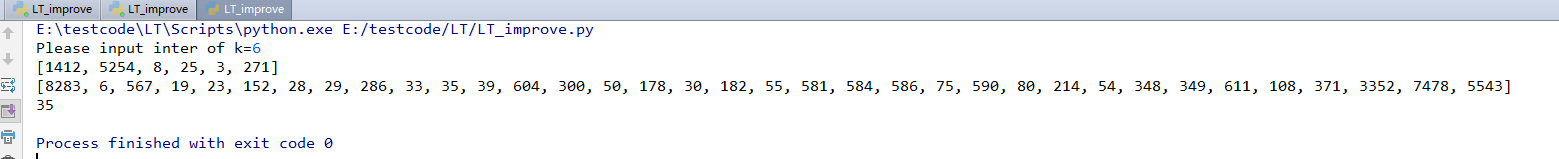交流学习资料共享欢迎入QQ群：955817470​​​​​​​展开全文社交网络 贪心算法
• 社交网络影响力最大化——线性阈值模型（LT模型）算法实现（Python实现） １、环境配置 环境配置：Win7 Pycharm Anaconda2 该算法每个节点的阈值设为 0.5 ２、LT传播模型算法实现 linear_threshold.py (L....
目录
１、环境配置
２、LT传播模型算法实现
３、LT传播模型算法测试
４、测试文件Wiki-Vote.txt数据

社交网络影响力最大化——线性阈值模型（LT模型）算法实现（Python实现）
１、环境配置
环境配置：Win7 Pycharm Anaconda2
该算法每个节点的阈值设为 0.5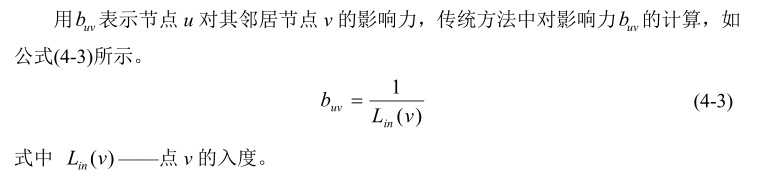２、LT传播模型算法实现
linear_threshold.py (LT传播模型算法)
# -*- coding: utf-8 -*-
"""
Implement linear threshold models
社交网络影响力最大化 传播模型——线性阈值（LT）模型算法实现
"""
import copy
import itertools
import random
import math
import networkx as nx

__all__ = ['linear_threshold']

#-------------------------------------------------------------------------
#  Some Famous Diffusion Models
#-------------------------------------------------------------------------

def linear_threshold(G, seeds, steps=0):           #LT线性阈值算法
"""
Parameters
----------
G : networkx graph                     #所有节点构成的图
The number of nodes.

seeds: list of nodes                   #子节点集
The seed nodes of the graph

steps: int                             #激活节点的层数（深度），当steps<=0时，返回子节点集能激活的所有节点
The number of steps to diffuse
When steps <= 0, the model diffuses until no more nodes
can be activated

Return
------
layer_i_nodes : list of list of activated nodes
layer_i_nodes: the seeds                  #子节点集
layer_i_nodes[k]: the nodes activated at the kth diffusion step   #该子节点集激活的节点集

Notes
-----
1. Each node is supposed to have an attribute "threshold".  If not, the
default value is given (0.5).    #每个节点有一个阈值，这里默认阈值为：0.5
2. Each edge is supposed to have an attribute "influence".  If not, the
default value is given (1/in_degree)  #每个边有一个权重值，这里默认为：1/入度

References
----------
 GranovetterMark. Threshold models of collective behavior.
The American journal of sociology, 1978.
"""

if type(G) == nx.MultiGraph or type(G) == nx.MultiDiGraph:
raise Exception( \
"linear_threshold() is not defined for graphs with multiedges.")

# make sure the seeds are in the graph
for s in seeds:
if s not in G.nodes():
raise Exception("seed", s, "is not in graph")

# change to directed graph
if not G.is_directed():
DG = G.to_directed()
else:
DG = copy.deepcopy(G)        # copy.deepcopy 深拷贝 拷贝对象及其子对象

# init thresholds
for n in DG.nodes():
if 'threshold' not in DG.node[n]:
DG.node[n]['threshold'] = 0.5
elif DG.node[n]['threshold'] > 1:
raise Exception("node threshold:", DG.node[n]['threshold'], \
"cannot be larger than 1")

# init influences
in_deg = DG.in_degree()       #获取所有节点的入度
for e in DG.edges():
if 'influence' not in DG[e][e]:
DG[e][e]['influence'] = 1.0 / in_deg[e]    #计算边的权重
elif DG[e][e]['influence'] > 1:
raise Exception("edge influence:", DG[e][e]['influence'], \
"cannot be larger than 1")

# perform diffusion
A = copy.deepcopy(seeds)
if steps <= 0:
# perform diffusion until no more nodes can be activated
return _diffuse_all(DG, A)
# perform diffusion for at most "steps" rounds only
return _diffuse_k_rounds(DG, A, steps)

def _diffuse_all(G, A):
layer_i_nodes = [ ]
layer_i_nodes.append([i for i in A])
while True:
len_old = len(A)
A, activated_nodes_of_this_round = _diffuse_one_round(G, A)
layer_i_nodes.append(activated_nodes_of_this_round)
if len(A) == len_old:
break
return layer_i_nodes

def _diffuse_k_rounds(G, A, steps):
layer_i_nodes = [ ]
layer_i_nodes.append([i for i in A])
while steps > 0 and len(A) < len(G):
len_old = len(A)
A, activated_nodes_of_this_round = _diffuse_one_round(G, A)
layer_i_nodes.append(activated_nodes_of_this_round)
if len(A) == len_old:
break
steps -= 1
return layer_i_nodes

def _diffuse_one_round(G, A):
activated_nodes_of_this_round = set()
for s in A:
nbs = G.successors(s)
for nb in nbs:
if nb in A:
continue
active_nb = list(set(G.predecessors(nb)).intersection(set(A)))
if _influence_sum(G, active_nb, nb) >= G.node[nb]['threshold']:
A.extend(list(activated_nodes_of_this_round))
return A, list(activated_nodes_of_this_round)

def _influence_sum(G, froms, to):
influence_sum = 0.0
for f in froms:
influence_sum += G[f][to]['influence']
return influence_sum


３、LT传播模型算法测试
test_linear_threshold.py（LT模型算法测试）
#!/usr/bin/env python
# coding=UTF-8                 #支持中文字符需要添加  coding=UTF-8
from nose.tools import *
from networkx import *
from linear_threshold import *
import time
"""Test Diffusion Models
----------------------------
"""
if __name__=='__main__':
start=time.clock()
datasets=[]
f=open("Wiki-Vote.txt","r")        #读取文件数据（边的数据）
rows=data.split('\n')
for row in rows:
split_row=row.split('\t')
name=(int(split_row),int(split_row))
datasets.append(name)            #将边的数据以元组的形式存放到列表中

G=networkx.DiGraph()               #建立一个空的有向图G
layers=linear_threshold(G,,2)     #调用LT线性阈值算法，返回子节点集和该子节点集的最大激活节点集
del layers[-1]
length=0
for i in range(len(layers)):
length =length+len(layers[i])
lengths=length-len(layers)       #获得子节点的激活节点的个数（长度）
end=time.clock()
#测试数据输出结果
print(layers)  #[, [33, 3, 6, 8, 55, 80, 50, 19, 54, 23, 75, 28, 29, 30, 35]]
print(lengths) #15
print('Running time: %s Seconds'%(end-start))  #输出代码运行时间


４、测试文件Wiki-Vote.txt数据
注释：测试文件Wiki-Vote.txt数据如下（每组数据代表图的有向边）
# FromNodeId ToNodeId
30 1412
30 3352
30 5254
30 5543
30 7478
3 28
3 30
3 39
3 54
3 108
3 152
3 178
3 182
3 214
3 271
3 286
3 300
3 348
3 349
3 371
3 567
3 581
3 584
3 586
3 590
3 604
3 611
3 8283
25 3
25 6
25 8
25 19
25 23
25 28
25 29
25 30
25 33
25 35
25 50
25 54
25 55
25 75
25 80
数据获取，交流学习资料共享欢迎入QQ群：955817470展开全文社交网络 线性阈值模型
• 对于影响力最大化问题，我以前写过几个blog 影响力最大化 IC模型+贪心算法 影响力最大化 模拟爆发(粗糙笔记) 影响力最大化 IC 蒙特卡洛模拟 贪心算法 影响力最大化 IMRank 我心中的最优算法 这篇文章采用CELF的算法...python 算法 图计算
• 个人对于影响力最大化这个问题本身比较感兴趣，这是我原来写过的链接： 影响力最大化 IMRank 我心中的最优算法 影响力最大化 模拟爆发(粗糙笔记) 影响力最大化 IC模型+贪心算法 这一偏还是基于贪心算法的IC模型，...python IC模型 蒙特卡洛模拟
• 对于影响力最大化问题，我以前写过两个blog 影响力最大化 IC模型+贪心算法 影响力最大化 模拟爆发(粗糙笔记) 但是，对于这两个方法都不是最优的： 对于IC模型 模型使用了贪心算法，然后遍历激活结点，每一次遍历将...算法 python
• 2、影响力最大化问题分类 3、社交网络影响力最大化作用 4、传播模型 4.1独立级联模型（Independent Cascade Model）简称 IC 模型 4.2线性阈值模型（Linear Threshold Model）简称LT模型 社交网络影响力最大化...社交网络 IC模型 LT模型
• 社交网络中基于位置的影响力最大化 摘要 这篇文章的目的是通过研究在LBSN平台中基于位置的影响最大化来实现O2O模式上的产品推广。随着O2O环境下存在的消费行为，传统的线上影响力扩散模型不能准确描述产品验收过程。...社交网络 算法
• 问题：找出图G中影响力最大的顶点。 注：结果不一定唯一。 输入 图G，以邻接表的形式。 点的标号从0到n-1，总共n个点，于是有n行输入。 第 i 行的输入为与编号 i-1 这个点相邻的点们的标号，以空格隔开...算法
• 某电信集团在资源信息管理上存在业务线程多，人员信息与其他系统内信息交叉多的问题，对人力部门来说，公司人员、岗位、薪酬、KPI等资源的调整指导没有实际的可视的参考，很多调整还是靠着人为的判断和操作来...商业智能 解决方案
• ## 4款最具影响力的自助式BI工具

千次阅读 多人点赞 2016-11-20 19:52:27
年报表开发领域的经验的，其最大优势在于分析速度的简单与快。凭借大数据引擎，用户只需在 dashboard 中拖拽分析的维度和指标 ， 自行完善可视的布局 ， 无需编辑代码和脚本 ， 就能呈现一个完整的数据报告 。 ...BI工具 数据分析jobs 互联网 云计算
• 社交影响力可以通过用户之间的社交huo'dong社交网络 算法
• 1999年，Gartner Group Inc公司提出了CRM概念...赋予企业更完善的客户交流能力，最大化客户的收益率。 直到今天 ，云计算的全球化使得传统CRM 软件已逐渐被Web CRM（又称为“在线CRM”、“托管型CRM”和“按需CRM...crm系统 客户管理软件 客户管理系统 客户关系管理
• Salesforce是全球云CRM的创始人。该公司成立于1999年3月，是按需CRM解决方案的全球领导者。Salesforce创始人和他的妻子以自己的...对于国内用户来说，流畅的体验和本地部署能力更受重视，因此受到中国的发展的影...客户关系管理系统 crm客户管理 teamface
• APP UI 自动测试的可行性」，「阅读原文」查看交流实录 「文末高能」 编辑 | 家辉 背 景 在这个科技时代，app 数量也是逐年递增，只要能想到，大多数都可以在相应的平台找到相关的 app! 那一款 ...
• ” 制造业及医疗保健行业为受科技影响最大的行业 综上可见，在后疫情时代，通过云计算、大数据、人工智能、物联网等新兴智能技术，紧锣密鼓地开展数字转型升级，实现自身更快更好地向前发展，是无数企业当前最为...人工智能 大数据 编程语言 物联网 机器学习
• 中国网民数量截止至11月已有8亿之多，互联网每天产生的数据也以PB规模增加，而今互联网显然已成为思想文化信息的集散地和社会舆论的放大镜，因此在2017年临近尾声之际，我们为您奉上刚出炉的品牌数字影响力盘点。...
• 2) 算法优化 由于上述论文中采用的算法是在传统的贪心算法上应用主题模型来进行社会网络的传播，其虽然有较好的近似比，但运行效率较低。...当传统的贪心算法根据边际影响力将第一个节点A加入种子节点后，将第一次计社会网络
• 建议重复上述证明步骤，用构造拉格朗日表达式，利用一阶条件来求解需求函数） 当 δ→∞\delta \rightarrow ∞δ→∞的时候，此时为完全互补效用函数，利用消费者为了效用最大化只会选择L型无差异曲线顶点消费的特征...
• 数据挖掘的第一步数据探索，包括汇总统计和可视，介绍了相关概念，并结合鸢尾花数据展示了如何用Python进行汇总统计量的计算以及常用的可视来帮助我们分析数据的性质。数据挖掘 python 数据
• Filter 之间不直接通信，在请求线程中会通过 RequestContext 来共享状态，它的内部是用 ThreadLocal 实现的，当然你也可以在 Filter之间使用 ThreadLocal 来收集自己需要的状态或数据。Zuul 中不同类型 filter 的...
• 不同的标准方法，对系统的评价结果会产生不同的影响，然而不幸的是，在数据标准方法的选择上，还没有通用的法则可以遵循。 标准（normalization）的目的： 在数据分析之前，我们通常需要先将数据标准...机器学习
• 一、什么是数字转型 数字转型是指通过对数字技术、...虽然数字转型主要用于商业环境，但它也影响其他组织，如政府、公共部门机构和组织，这些组织通过利用一项或多项现有和新兴技术，参与解决诸如污染和人口老
• 这里主要讲连续型特征归一的常用方法。 连续型特征还有一种处理方式是，先分桶/分箱（如等频/等距的分）[待写]进行离散后再使用离散数据的处理方法。 离散数据处理参考[数据预处理：独热编码（One-Hot ...
• ## 正态分布及matlab实现

万次阅读 多人点赞 2014-06-03 09:58:48
正态分布（Normal distribution）又名高斯分布（Gaussian distribution），是一个在数学、物理及工程等领域都非常重要的概率分布，在统计学的许多方面有着重大的影响力。 若随机变量X服从一个数学期望为μ、标准...
• ## 大屏数据可视化设计指南

万次阅读 多人点赞 2019-01-03 14:25:31
把相对复杂、抽象的数据通过可视的方式以人们更易理解的形式展示出来的一系列手段叫做数据可视，数据可视是为了更形象地表达数据内在的信息和规律，促进数据信息的传播和应用。 在当前新技术支持下，数据可视......